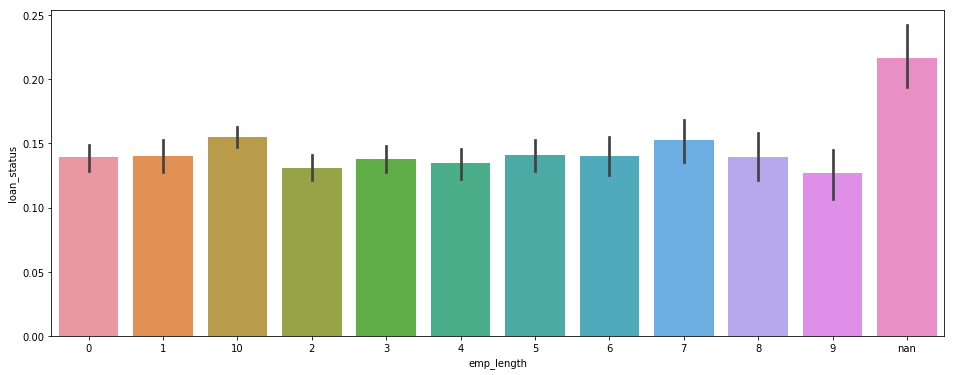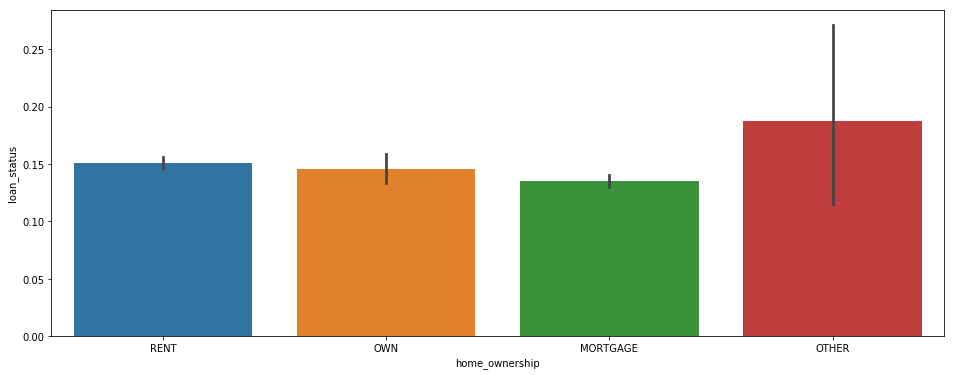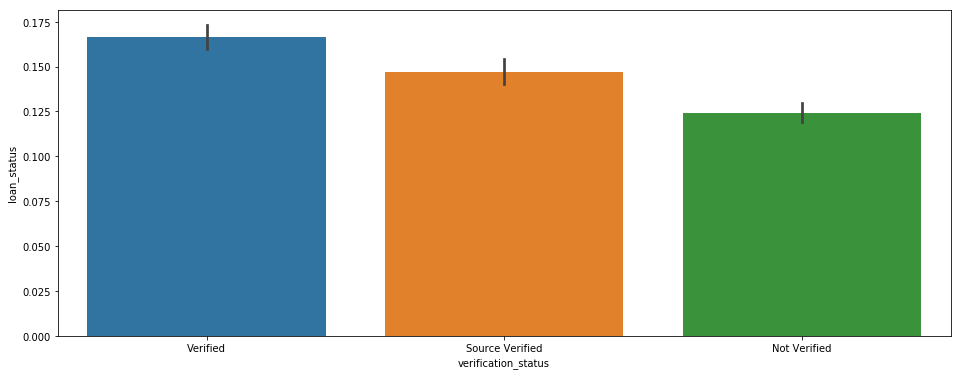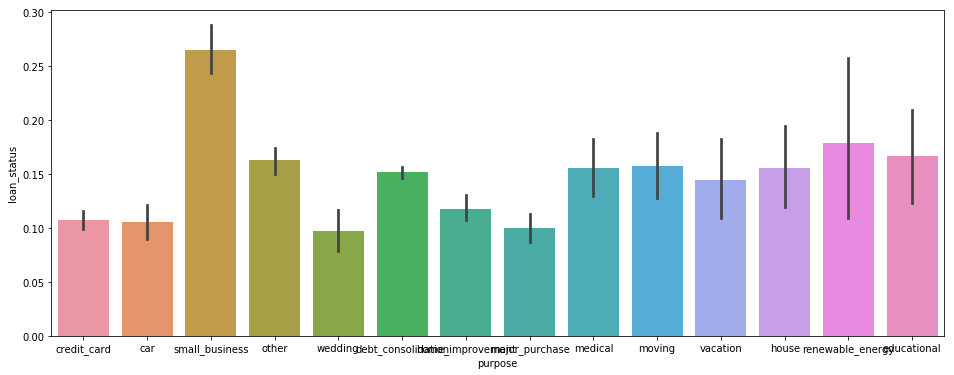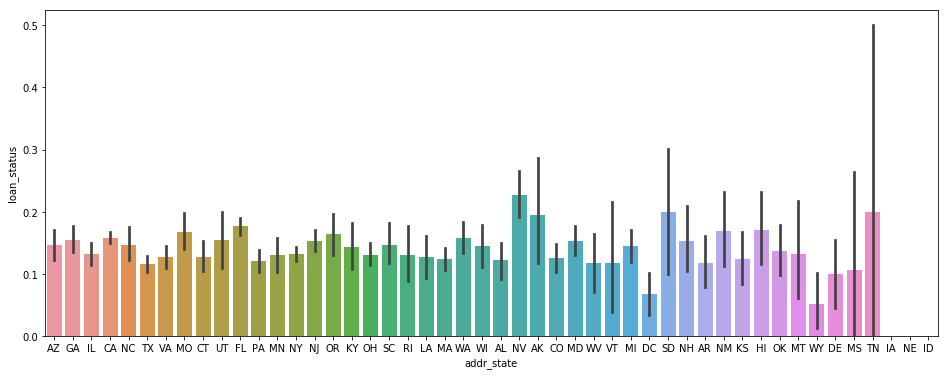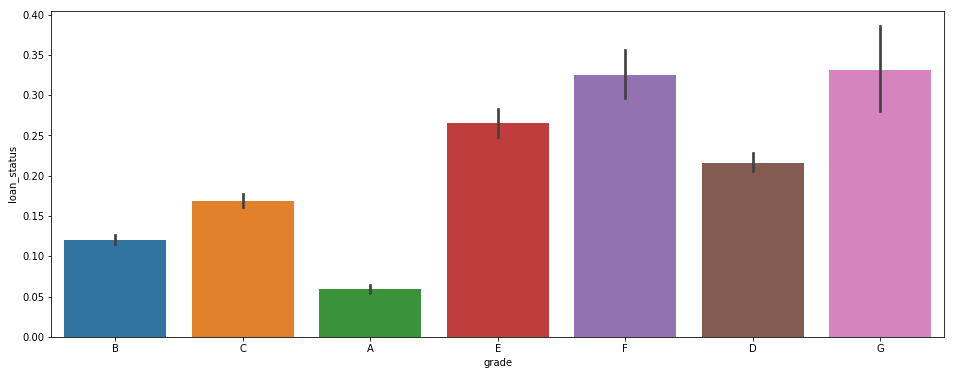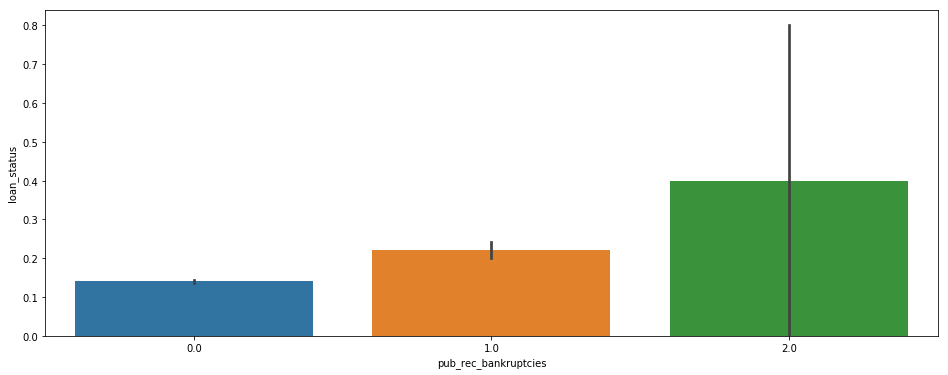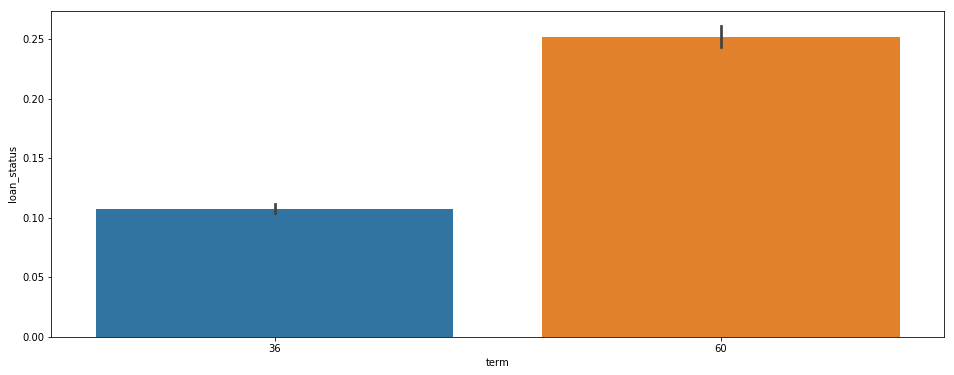# Gramener Case Study¶

Gramener is the largest online loan marketplace, facilitating personal loans, business loans, and financing of medical procedures. Borrowers can easily access lower interest rate loans through a fast online interface. Like most other lending companies, lending loans to ‘risky’ applicants is the largest source of financial loss (called credit loss).

The credit loss is the amount of money lost by the lender when the borrower refuses to pay or runs away with the money owed. In other words, borrowers who default cause the largest amount of loss to the lenders. In this case, the customers labelled as 'charged-off' are the 'defaulters'. If one is able to identify these risky loan applicants, then such loans can be reduced thereby cutting down the amount of credit loss. Identification of such applicants using EDA is the aim of this case study.

In other words, the company wants to understand the driving factors (or driver variables) behind loan default, i.e. the variables which are strong indicators of default. The company can utilise this knowledge for its portfolio and risk assessment.

In this case study, we will apply EDA (Exploratory Data Analysis) Techniques to develop understanding of risk analytics and finacial service. With this EDA, we will try to understand how to minimise risk of loosing money while lending money to customer.

Gramener consumer finance company which specialises in lending various types of loans to urban customers. When the company receives a loan application, the company has to make a decision for loan approval based on the applicant’s profile. Two types of risks are associated with the bank’s decision:

1. If the applicant is likely to repay the loan, then not approving the loan results in a loss of business to the company

2. If the applicant is not likely to repay the loan, i.e. he/she is likely to default, then approving the loan may lead to a financial loss for the company

The data given in the dataset contains the information about past loan applicants and whether they ‘defaulted’ or not. The aim is to identify patterns which indicate if a person is likely to default, which may be used for taking actions such as denying the loan, reducing the amount of loan, lending (to risky applicants) at a higher interest rate, etc.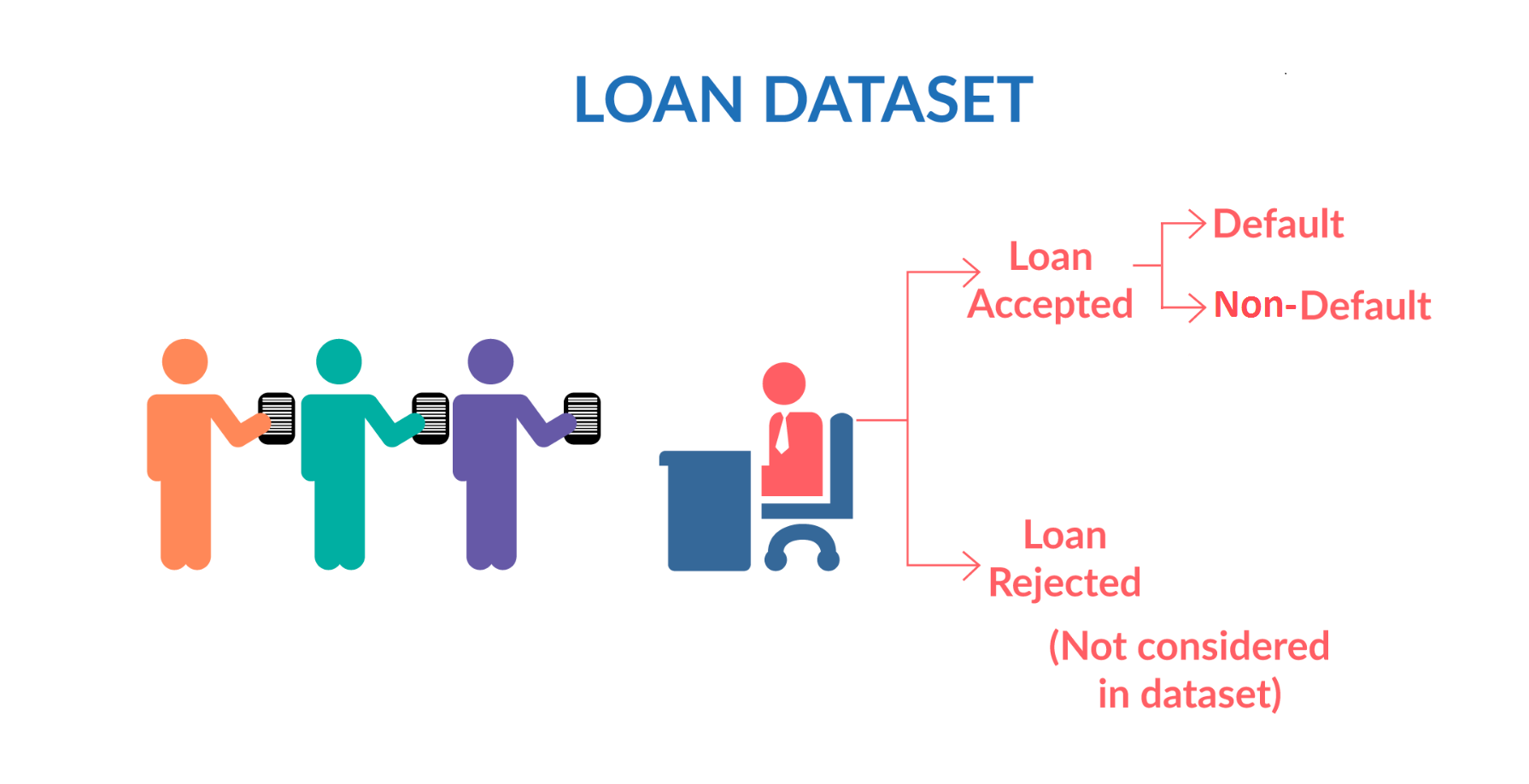When a person applies for a loan, there are two types of decisions that could be taken by the company:

Loan accepted: If the company approves the loan, there are 3 possible scenarios described below:

1. Fully paid: Applicant has fully paid the loan (the principal and the interest rate)

2. Current: Applicant is in the process of paying the instalments, i.e. the tenure of the loan is not yet completed. These candidates are not labelled as 'defaulted'.

3. Charged-off: Applicant has not paid the instalments in due time for a long period of time, i.e. he/she has defaulted on the loan

Loan rejected: The company had rejected the loan (because the candidate does not meet their requirements etc.). Since the loan was rejected, there is no transactional history of those applicants with the company and so this data is not available with the company (and thus in this dataset)

In :
## Import Python Modeules
import pandas as pd
import numpy as np
import matplotlib.pyplot as plt
import seaborn as sns


Next we will load the loan csv and then do the following

1) find out which all columns are having null/NaN values, find all these columns and drop them as they dont give any inputs.

2) use the null value dataframe to find which columns are null and filter out them from main data.

In :
loan_data = pd.read_csv('./loan.csv',low_memory=False)
print('size of data with id as unique column {}'.format(loan_data.id.count()))

# print(loan_data.isnull().sum()/len(loan_data.index))
nullSeries = loan_data.isnull().sum()/len(loan_data.index)
null_data = pd.DataFrame(nullSeries,columns=['nullindex'])
nonNullCol = list(null_data.loc[null_data.nullindex!=1.0].index)
loan_data = loan_data[nonNullCol]

print(loan_data.shape)

size of data with id as unique column 39717
(39717, 57)

Out:
id member_id loan_amnt funded_amnt funded_amnt_inv term int_rate installment grade sub_grade ... next_pymnt_d last_credit_pull_d collections_12_mths_ex_med policy_code application_type acc_now_delinq chargeoff_within_12_mths delinq_amnt pub_rec_bankruptcies tax_liens
0 1077501 1296599 5000 5000 4975.0 36 months 10.65% 162.87 B B2 ... NaN May-16 0.0 1 INDIVIDUAL 0 0.0 0 0.0 0.0
1 1077430 1314167 2500 2500 2500.0 60 months 15.27% 59.83 C C4 ... NaN Sep-13 0.0 1 INDIVIDUAL 0 0.0 0 0.0 0.0
2 1077175 1313524 2400 2400 2400.0 36 months 15.96% 84.33 C C5 ... NaN May-16 0.0 1 INDIVIDUAL 0 0.0 0 0.0 0.0
3 1076863 1277178 10000 10000 10000.0 36 months 13.49% 339.31 C C1 ... NaN Apr-16 0.0 1 INDIVIDUAL 0 0.0 0 0.0 0.0
4 1075358 1311748 3000 3000 3000.0 60 months 12.69% 67.79 B B5 ... Jun-16 May-16 0.0 1 INDIVIDUAL 0 0.0 0 0.0 0.0

5 rows × 57 columns

In :
d = pd.DataFrame(loan_data.isnull().sum()/len(loan_data.index),columns=['nullper'])
d = d.loc[d.nullper > 0]

e = pd.DataFrame(loan_data[loan_data.loan_status == 'Charged Off'].isnull().sum()/len(loan_data[loan_data.loan_status == 'Charged Off'].index),columns=['co_nullper'])
e = e.loc[e.co_nullper > 0]

pd.concat([d,e],axis=1)

Out:
nullper co_nullper
emp_title 0.061913 0.086014
emp_length 0.027066 0.040519
desc 0.325805 0.320242
title 0.000277 0.000355
mths_since_last_delinq 0.646625 0.619335
mths_since_last_record 0.929854 0.897281
revol_util 0.001259 0.002843
last_pymnt_d 0.001788 0.012618
next_pymnt_d 0.971297 1.000000
last_credit_pull_d 0.000050 0.000178
collections_12_mths_ex_med 0.001410 0.001066
chargeoff_within_12_mths 0.001410 0.001066
pub_rec_bankruptcies 0.017549 0.020970
tax_liens 0.000982 0.000178

Now we will start data cleaning column wise and since the below columns have large % of null values.

1) 'mths_since_last_record' --> 92% null values,

2) 'next_pymnt_d' --> 97% null values

variables with less business significance 3) member_id --> because its a random number and we already have id as unique key. No inputs for making Charged off decisions

4) funded_amnt_inv --> The loan is funded, and investers are not going to get money back :)

6) issue_d --> the loan is already funded

7) zip_code --> Unable to process the data to a meaning form as it requires lot of patience.

8) out_prncp --> It kind of gives information to current loan rather than charged off cases.

9) out_prncp_inv --> It kind of gives information to current loan rather than charged off cases.

10) total_pymnt_inv --> redundant columns WRT to total_pymnt

11) total_rec_prncp, total_rec_int --> It kind of gives information to current loan rather than charged off cases.

replace the text columns with some knows strings instead of dropping the rows for it. then for the remaining columns which have null values we can drop the corresponding rows.

In :
loan_data = loan_data.drop(['mths_since_last_record','next_pymnt_d','member_id','funded_amnt_inv','sub_grade'\
,'issue_d','zip_code','out_prncp','out_prncp_inv','total_pymnt_inv','total_rec_prncp'\
, 'total_rec_int' ], axis=1)


Analysis to check for mths_since_last_delinq releaved that for the column the nulls values are for cases charged off, hence replacing that column with std or median should be fine. Prefering std value so that we dont put too much penalty on the 4% null values which are in non-default cases.

In :
a = loan_data[loan_data.loan_status == 'Charged Off'].mths_since_last_delinq.dropna()
print(a.describe([0.1,0.25,0.75,0.9,0.95,0.97,0.99]))

## identify lower and upper bound and remove outliers
IQR = a.quantile(0.75) - a.quantile(0.25)
step = 1.0 * IQR
lowerbound = 0 if (a.quantile(0.25) - step) < 0 else (a.quantile(0.25) - step)
upperbound = a.quantile(0.75) + step
print(lowerbound)
print(upperbound)

a = a[a < upperbound]
plt.figure(figsize=(17,5))
plt.subplot(131)
sns.distplot(a)
plt.subplot(132)
sns.boxplot(y = a)
plt.subplot(133)
plt.hist(a)
#plt.yscale('log')
plt.show()

a.describe([0.1,0.25,0.75,0.9,0.95,0.97,0.99])

count    2142.000000
mean       36.157330
std        22.679333
min         0.000000
10%         7.000000
25%        17.000000
50%        34.000000
75%        53.000000
90%        71.000000
95%        76.000000
97%        78.770000
99%        81.000000
max       107.000000
Name: mths_since_last_delinq, dtype: float64
0
89.0

/anaconda3/lib/python3.6/site-packages/matplotlib/axes/_axes.py:6462: UserWarning: The 'normed' kwarg is deprecated, and has been replaced by the 'density' kwarg.
warnings.warn("The 'normed' kwarg is deprecated, and has been "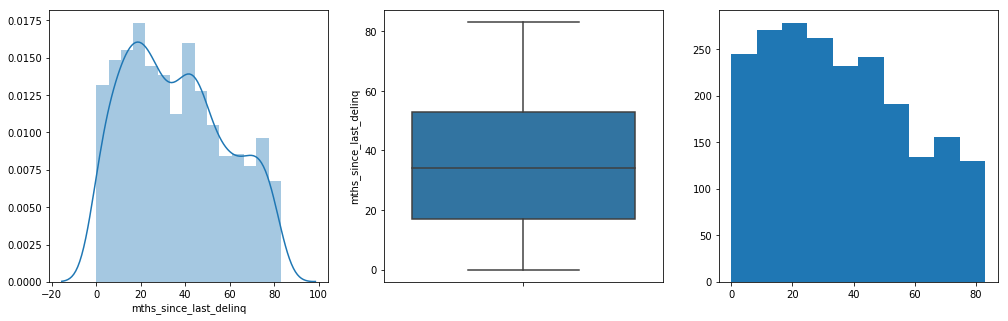Out:
count    2140.000000
mean       36.095794
std        22.599832
min         0.000000
10%         7.000000
25%        17.000000
50%        34.000000
75%        53.000000
90%        70.100000
95%        76.000000
97%        78.000000
99%        81.000000
max        83.000000
Name: mths_since_last_delinq, dtype: float64

Now we will start conditioning/value treatments for the respective columns after looking sample data from each column, like remove the % from float percent values, impute values And specifically for empoyee experience convert the categories into 10 buckets

In :
loan_data.mths_since_last_delinq.fillna(22.5,inplace=True)
loan_data.emp_title.fillna('unknown_emp_title', inplace=True)
loan_data.desc.fillna('unknow_desc',inplace=True)
loan_data.title.fillna('unknow_title',inplace=True)

In :
type(loan_data['emp_length'])

Out:
str
In :
loan_data["emp_length"] = loan_data["emp_length"].astype(str)
# treating   emp_length as categorical by converting to nominal variable
loan_data["emp_length"] = loan_data["emp_length"].apply(lambda x: '0' if (x == 'n/a' or x == '< 1 year') else x)
loan_data["emp_length"] = loan_data["emp_length"].apply(lambda x: x.replace("years", "").replace("year", "").replace("+","").replace(">","").strip())

print('rows having number of null values {}'.format(set(loan_data.isnull().sum(axis=1))))
print('columns having number of null values {}'.format(set(loan_data.isnull().sum())))
loan_data = loan_data.dropna()

print(loan_data.shape)
d = pd.DataFrame(loan_data.isnull().sum()/len(loan_data.index),columns=['nullper'])
d = d.loc[d.nullper > 0]

e = pd.DataFrame(loan_data[loan_data.loan_status == 'Charged Off'].isnull().sum()/len(loan_data[loan_data.loan_status == 'Charged Off'].index),columns=['co_nullper'])
e = e.loc[e.co_nullper > 0]

pd.concat([d,e],axis=1)

rows having number of null values {0, 1, 2, 3, 4}
columns having number of null values {0, 2, 71, 39, 50, 56, 697}
(38900, 45)

Out:
nullper co_nullper
In :
#uncomment this code to see sample value for each column.
# cols = loan_data.columns.values
# for colName in cols:

loan_data['term'] = loan_data.term.apply(lambda x: int(x[0:3]))
loan_data['int_rate'] = loan_data.int_rate.apply(lambda x: float(x.strip('%')))
loan_data['revol_util'] = loan_data.revol_util.apply(lambda x: float(x.strip('%')))

print(loan_data.shape)

(38900, 45)

Out:
id loan_amnt funded_amnt term int_rate installment grade emp_title emp_length home_ownership ... last_pymnt_amnt last_credit_pull_d collections_12_mths_ex_med policy_code application_type acc_now_delinq chargeoff_within_12_mths delinq_amnt pub_rec_bankruptcies tax_liens
0 1077501 5000 5000 36 10.65 162.87 B unknown_emp_title 10 RENT ... 171.62 May-16 0.0 1 INDIVIDUAL 0 0.0 0 0.0 0.0
1 1077430 2500 2500 60 15.27 59.83 C Ryder 0 RENT ... 119.66 Sep-13 0.0 1 INDIVIDUAL 0 0.0 0 0.0 0.0
2 1077175 2400 2400 36 15.96 84.33 C unknown_emp_title 10 RENT ... 649.91 May-16 0.0 1 INDIVIDUAL 0 0.0 0 0.0 0.0
3 1076863 10000 10000 36 13.49 339.31 C AIR RESOURCES BOARD 10 RENT ... 357.48 Apr-16 0.0 1 INDIVIDUAL 0 0.0 0 0.0 0.0
4 1075358 3000 3000 60 12.69 67.79 B University Medical Group 1 RENT ... 67.79 May-16 0.0 1 INDIVIDUAL 0 0.0 0 0.0 0.0

5 rows × 45 columns

In :
invSet = ["","n/a","N/A"]
cols = loan_data.columns.values
for colName in cols:
#     print('column contain invalid values {0}:{1}'.format(colName,loan_data.loc[loan_data[colName].isin(invSet)].empty))

print('probable Categorical values')
print('probable Continuous values')

probable Categorical values
['grade', 'emp_title', 'emp_length', 'home_ownership', 'verification_status', 'loan_status', 'pymnt_plan', 'url', 'desc', 'purpose', 'title', 'addr_state', 'earliest_cr_line', 'initial_list_status', 'last_pymnt_d', 'last_credit_pull_d', 'application_type']

probable Continuous values
['id', 'loan_amnt', 'funded_amnt', 'term', 'delinq_2yrs', 'inq_last_6mths', 'open_acc', 'pub_rec', 'revol_bal', 'total_acc', 'policy_code', 'acc_now_delinq', 'delinq_amnt']

['int_rate', 'installment', 'annual_inc', 'dti', 'mths_since_last_delinq', 'revol_util', 'total_pymnt', 'total_rec_late_fee', 'recoveries', 'collection_recovery_fee', 'last_pymnt_amnt', 'collections_12_mths_ex_med', 'chargeoff_within_12_mths', 'pub_rec_bankruptcies', 'tax_liens']



1) Again use the categorical value first and then check if each category is a single constant value and then we can plan to exclude that as that will not give us any information

2) Build a dataframe on the univariate analysis for all the comtinuous variables, and then check if the columns is having single value like 0.0 or 0 and drop them as they dont give us information for analysis

3) Filter out the desc, url , title and emp_title as they can be considered for sentiment analysis kind of overwork here.

In :
# seeing from popular categories for which we can check on the analysis.
catColList = list()
if(eachCat not in ['desc','url','title','emp_title']):
#         print('{0} : {1}'.format(eachCat,set(loan_data[eachCat].unique())))
if(len(loan_data[eachCat].unique()) > 1):
catColList.append(eachCat)

#TODO for each continous column create the table of description and append the values to check how the data is changing per col
quantRange = [0.1,0.25,0.75,0.9,0.95,0.97,0.99]
condf = pd.DataFrame()
if(eachCol not in ['id','member_id']):
a = pd.DataFrame(loan_data[[eachCol]].describe(quantRange))[1:]#excluding the count as we are aware of that metric
condf = pd.concat([a,condf], axis=1)

a = pd.DataFrame(loan_data[[eachCol]].describe(quantRange))[1:]#excluding the count as we are aware of that metric
condf = pd.concat([a,condf], axis=1)

#for univariate analysis on which has constant value might not have any inputs to be given for EDA (but can be considered for modelling)
condf.to_csv('./univariate_column_meta_data.csv')
nunique = condf.apply(pd.Series.nunique)
cols_to_drop = nunique[nunique == 1].index
print('columns having unique/single value {}'.format(cols_to_drop))
condf = condf.drop(cols_to_drop, axis=1)

reqColList = list(['id']) + list(catColList) + list(condf.columns.values)
loan_data = loan_data[reqColList]
loan_data.to_csv('./loan_cleanedup.csv')
print(loan_data.columns.values)

columns having unique/single value Index(['tax_liens', 'chargeoff_within_12_mths', 'collections_12_mths_ex_med',
'delinq_amnt', 'acc_now_delinq'],
dtype='object')
'last_credit_pull_d' 'pub_rec_bankruptcies' 'last_pymnt_amnt'
'collection_recovery_fee' 'recoveries' 'total_rec_late_fee' 'total_pymnt'
'revol_util' 'mths_since_last_delinq' 'dti' 'annual_inc' 'installment'
'int_rate' 'policy_code' 'total_acc' 'revol_bal' 'pub_rec' 'open_acc'
'inq_last_6mths' 'delinq_2yrs' 'term' 'funded_amnt' 'loan_amnt']

Out:
id grade emp_length home_ownership verification_status loan_status purpose addr_state earliest_cr_line last_pymnt_d ... policy_code total_acc revol_bal pub_rec open_acc inq_last_6mths delinq_2yrs term funded_amnt loan_amnt
0 1077501 B 10 RENT Verified Fully Paid credit_card AZ Jan-85 Jan-15 ... 1 9 13648 0 3 1 0 36 5000 5000
1 1077430 C 0 RENT Source Verified Charged Off car GA Apr-99 Apr-13 ... 1 4 1687 0 3 5 0 60 2500 2500
2 1077175 C 10 RENT Not Verified Fully Paid small_business IL Nov-01 Jun-14 ... 1 10 2956 0 2 2 0 36 2400 2400
3 1076863 C 10 RENT Source Verified Fully Paid other CA Feb-96 Jan-15 ... 1 37 5598 0 10 1 0 36 10000 10000
4 1075358 B 1 RENT Source Verified Current other OR Jan-96 May-16 ... 1 38 27783 0 15 0 0 60 3000 3000

5 rows × 33 columns

In :
loan_data.columns

Out:
Index(['id', 'grade', 'emp_length', 'home_ownership', 'verification_status',
'last_pymnt_d', 'last_credit_pull_d', 'pub_rec_bankruptcies',
'last_pymnt_amnt', 'collection_recovery_fee', 'recoveries',
'total_rec_late_fee', 'total_pymnt', 'revol_util',
'mths_since_last_delinq', 'dti', 'annual_inc', 'installment',
'int_rate', 'policy_code', 'total_acc', 'revol_bal', 'pub_rec',
'open_acc', 'inq_last_6mths', 'delinq_2yrs', 'term', 'funded_amnt',
'loan_amnt'],
dtype='object')
In :
loan_data['loan_status'].value_counts()

Out:
Fully Paid     32336
Charged Off     5424
Current         1140
Name: loan_status, dtype: int64

## We can see that, most of the loans comprises of fully_paid status, while there is some chunk which is current running loans. We can not analyse based on current loans as they have not yet defaulted so lets get rid of them.¶

In :
loan_data = loan_data[loan_data['loan_status'] != 'Current']


# Univariate Analysis¶

1) Perform the univariate (for categorical and continous) indivdual columns, like removal of outliers, finding the tuning the mean and 50th quantile difference

2) Derrive new columns as well as and when required.

In :
#create some common functions for performing univariate analysis
#For funded_amount
def outlierranges(loan_data,col_name,exp):
## identify lower and upper bound and remove outliers
IQR = loan_data[col_name].quantile(0.75) - loan_data[col_name].quantile(0.25)
step = exp * IQR
lowerbound = 0 if (loan_data[col_name].quantile(0.25) - step) < 0 else (loan_data[col_name].quantile(0.25) - step)
upperbound = loan_data[col_name].quantile(0.75) + step
print('lower bound ', lowerbound)
print('upper bound ', upperbound)
return lowerbound,upperbound

def plotContinousVar(loan_data,col_name,logScale):
plt.figure(figsize=(20,5))
plt.subplot(131)
sns.distplot(loan_data[col_name])
plt.subplot(132)
sns.boxplot(y = loan_data[col_name])
plt.subplot(133)
plt.hist(loan_data[col_name])
if(logScale == True):
plt.yscale('log')
plt.show()

def getDefaultPercentageDF(loan_data, column_name):
#calculate total count per category
count_df = loan_data[['id',each]].groupby(each, as_index=False)\
.count()\
.sort_values(by='id',ascending=False)
count_df = count_df.rename(index=str,columns={'id':'total_count'})

#calculate charged off count per category
co_count_df = loan_data[['id','loan_status',each]].loc[loan_data.loan_status ==1]\
.groupby([each,'loan_status'],as_index=False)\
.count()
co_count_df = co_count_df.rename(index=str,columns={'id':'co_count'})

#merge the two categories and calculate the derived column for charged off % and see which is having high variance
final_df = pd.merge(count_df,co_count_df,on=each)
final_df['default %'] = round((final_df.co_count/final_df.total_count)*100,2)
return count_df,final_df

In :
## Lets convert loan_status to numeric field for summarization
loan_data['loan_status'] = loan_data['loan_status'].apply(lambda x: 0 if x=='Fully Paid' else 1)

# converting loan_status to integer type
loan_data['loan_status'] = loan_data['loan_status'].apply(lambda x: pd.to_numeric(x))

# summarising the values
loan_data['loan_status'].value_counts()

Out:
0    32336
1     5424
Name: loan_status, dtype: int64

Start Anayzing the categorical variables, but first doing a coutn plot of loans applied for each category compared to the charged off % of each category.

While analysing the categorical column, we see some categories in count plot and some categories in missing default %plot as the reason would be that they are not applicable for "charged off" cases

In :
cat_col_list = ['emp_length', 'home_ownership', 'verification_status', 'purpose','addr_state', 'grade',\
'pub_rec_bankruptcies','term','pub_rec','policy_code','delinq_2yrs','open_acc','total_acc']

for each in cat_col_list:
count_df, final_df = getDefaultPercentageDF(loan_data,each)

#plot to understand where the maximum loans are lended/funded
plt.figure(figsize=(20,5))
plt.subplot(121)
sns.pointplot(x=each, y="total_count", data=count_df)
plt.xticks(rotation=90)

plt.subplot(122)
sns.pointplot(x=each, y="default %", data=final_df)
plt.xticks(rotation=90)

plt.show()
print('--------------------------------------------------------------------------------------------------------------------')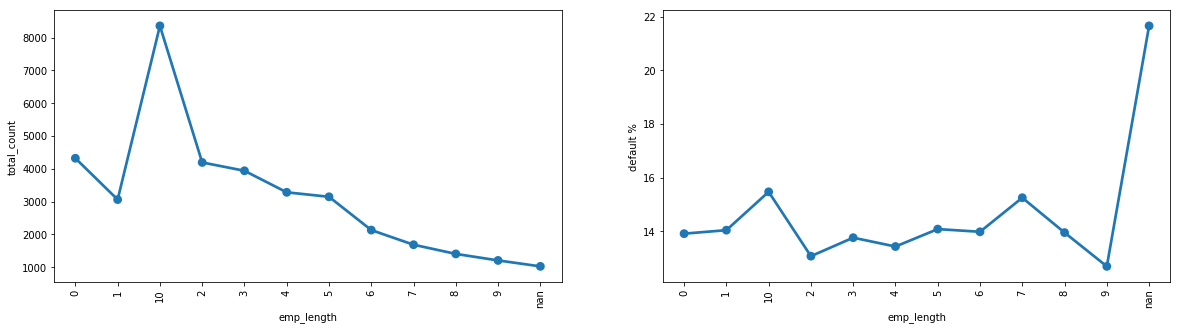--------------------------------------------------------------------------------------------------------------------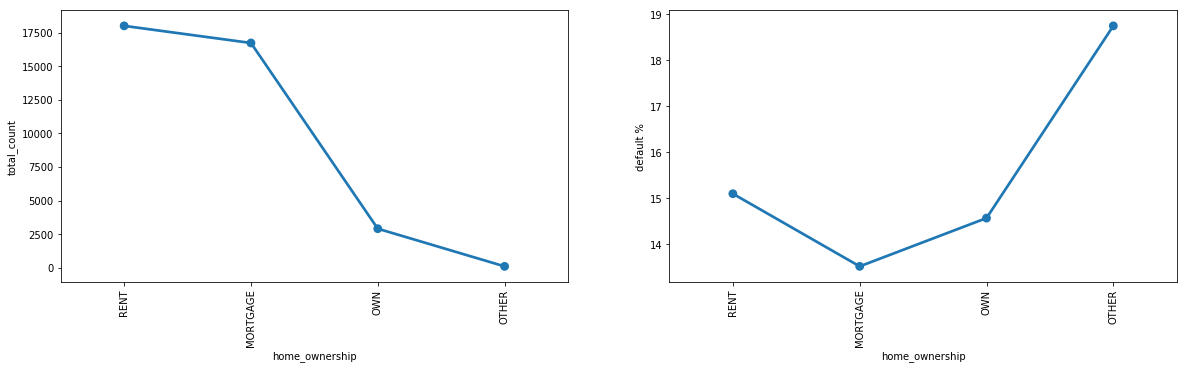--------------------------------------------------------------------------------------------------------------------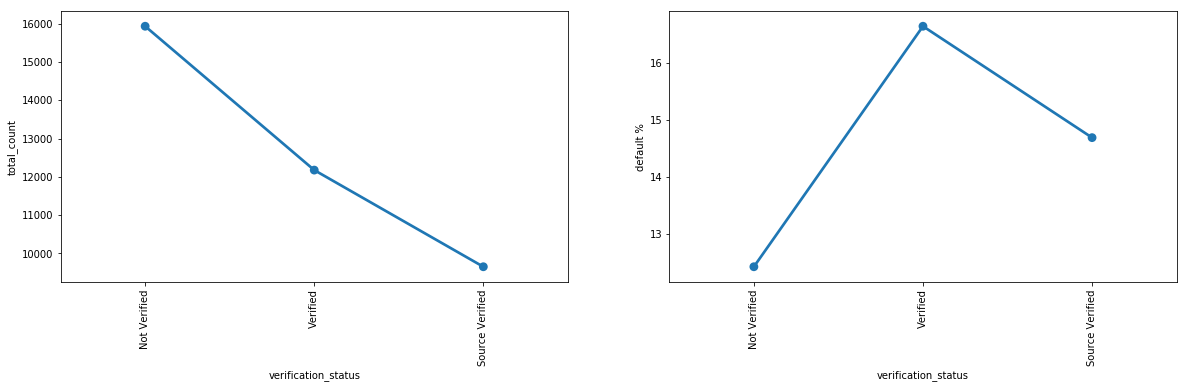--------------------------------------------------------------------------------------------------------------------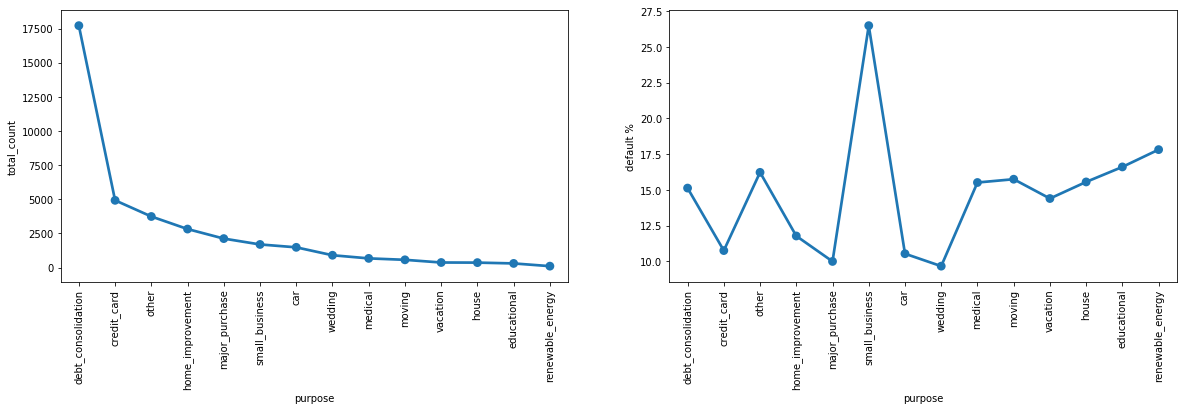--------------------------------------------------------------------------------------------------------------------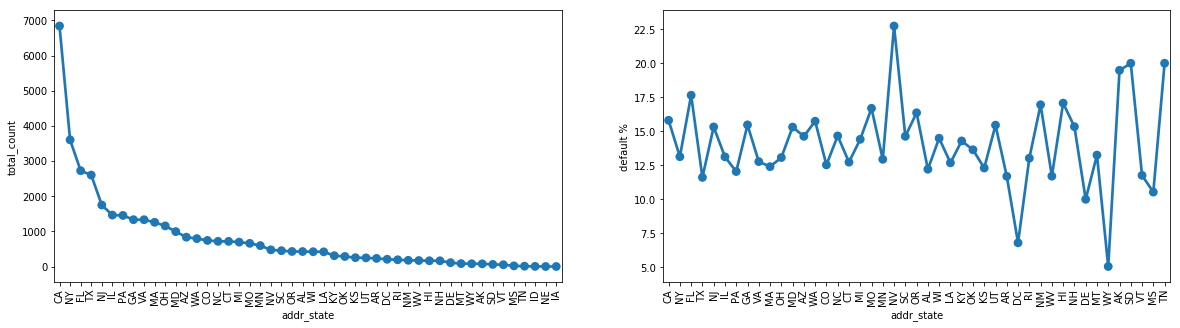--------------------------------------------------------------------------------------------------------------------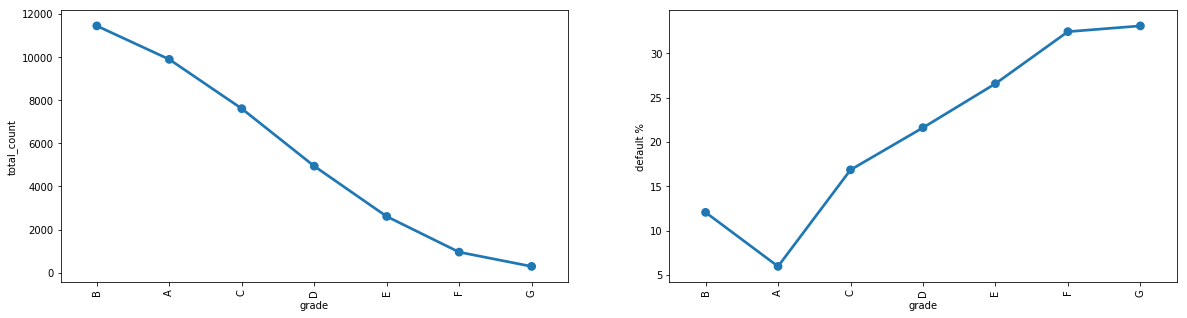--------------------------------------------------------------------------------------------------------------------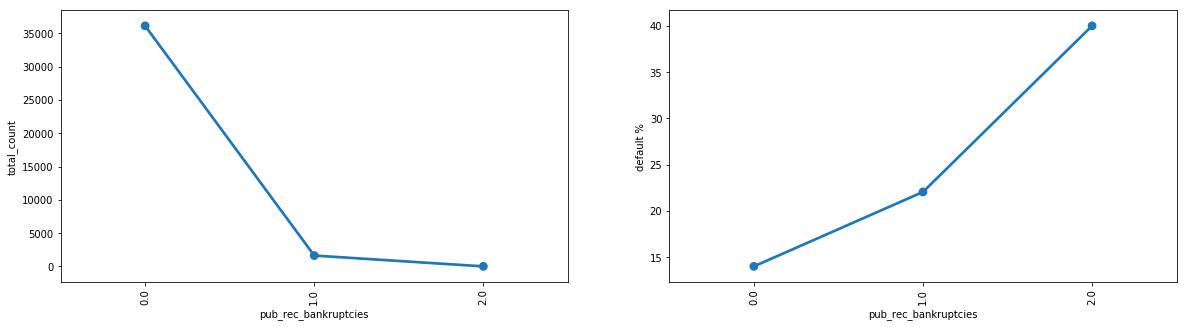--------------------------------------------------------------------------------------------------------------------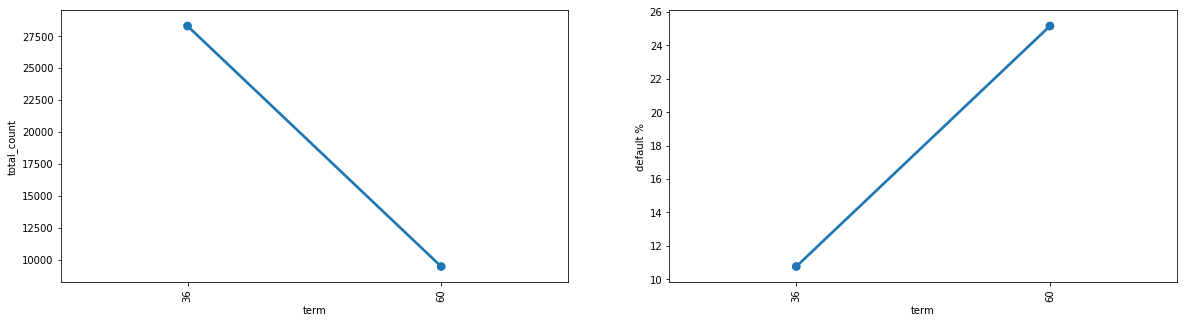--------------------------------------------------------------------------------------------------------------------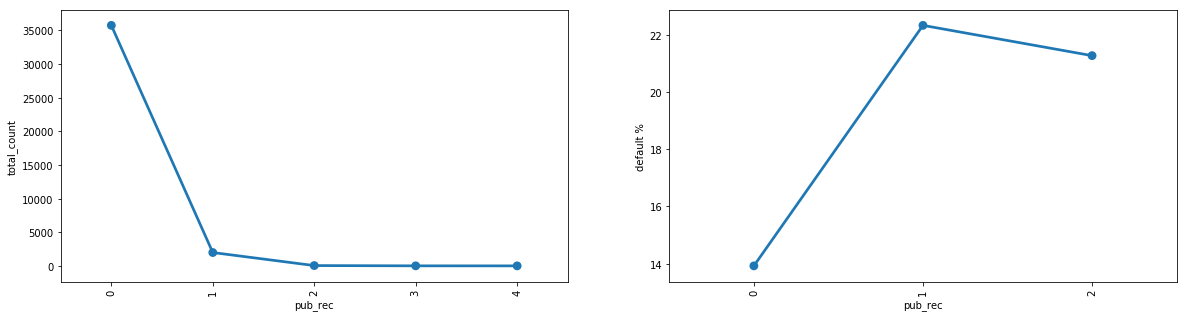--------------------------------------------------------------------------------------------------------------------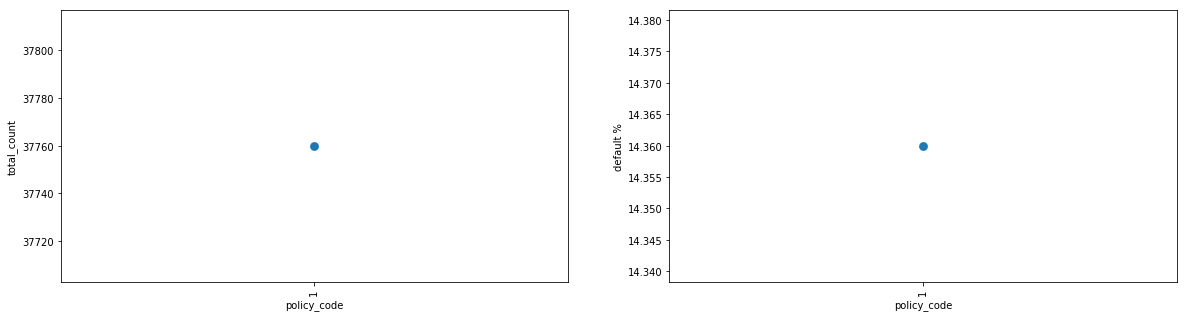--------------------------------------------------------------------------------------------------------------------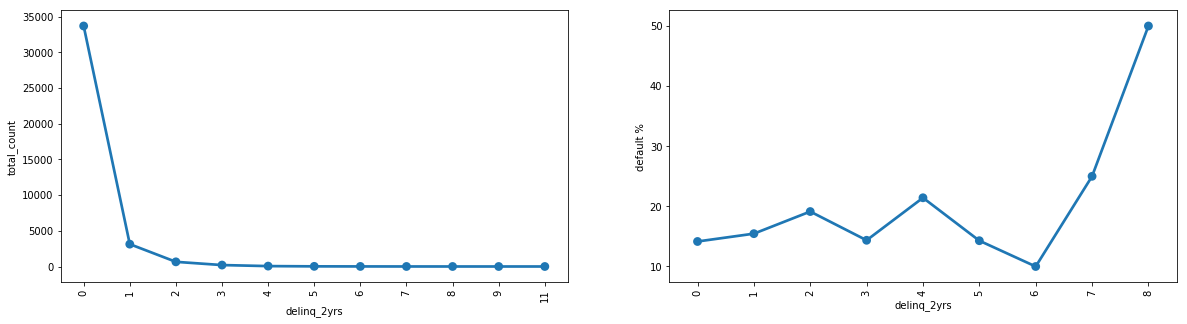--------------------------------------------------------------------------------------------------------------------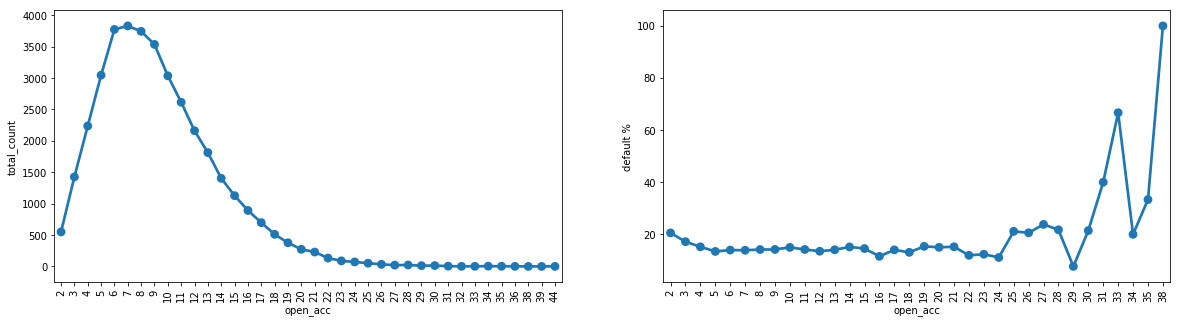--------------------------------------------------------------------------------------------------------------------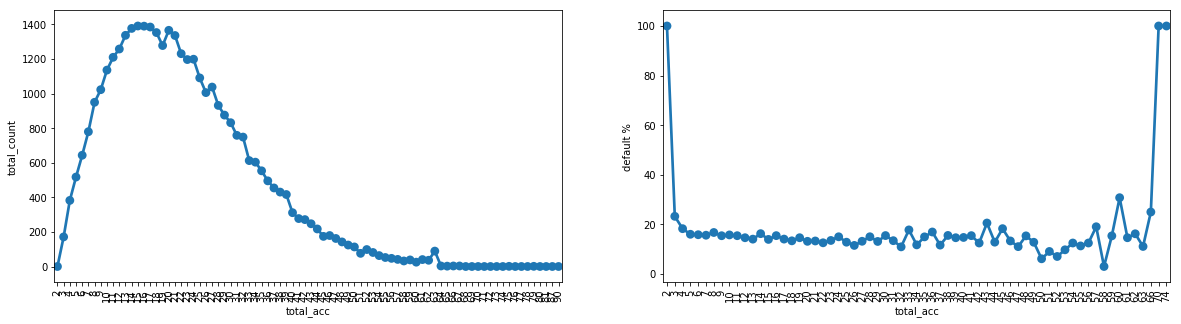--------------------------------------------------------------------------------------------------------------------

In :
# Lets plot default rates across various categorical features of the loan to understand the impact

for each in cat_col_list:
plt.figure(figsize=(16, 6))
sns.barplot(x=each, y='loan_status', data=loan_data)
plt.show()